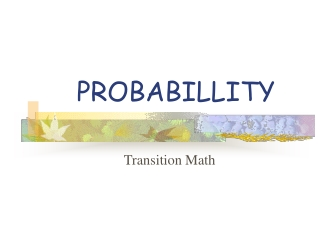DownloadDownload PresentationPROBABILLITY

# PROBABILLITY

Download Presentation## PROBABILLITY

- - - - - - - - - - - - - - - - - - - - - - - - - - - E N D - - - - - - - - - - - - - - - - - - - - - - - - - - -
##### Presentation Transcript

1. PROBABILLITY Transition Math

2. What is Probability? • Probability is a number from 0 to 1 that tells you how likely something is to happen. • Probability can be either theoretical or experimental.

3. THEORETICAL Theoretical probability can be found without doing and experiment. EXPERIMENTAL Experimental probability is found by repeating an experiment and observing the outcomes. Probability

4. HEADS TAILS THEORETICAL PROBABILITY • I have a quarterMy quarter has a heads side and a tails side • Since my quarter has only 2 sides, there are only 2 possible outcomes when I flip it. It will either land on heads, or tails

5. HEADS TAILS THEORETICAL PROBABILITY • When I flip my coin, the probability that my coin will land on heads is 1 in 2 • What is the probability that my coin will land on tails??

6. HEADS TAILS Theoretical Probability Right!!! There is a 1 in 2 probability that my coin will land on tails!!! • A probability of 1 in 2 can be written in three ways: • As a fraction: ½ • As a decimal: .50 • As a percent: 50%

7. A A A A Theoretical probability When I spin this spinner, I have a 1 in 4 chance of landing on the section with the red A in it.

8. A A A A Theoretical Probability A 1 in 4 chance can be written 3 ways: • As a fraction: ¼ • As a decimal: .25 • As a percent: 25%

9. Theoretical Probability I have three marbles in a bag. • I am going to take 1 marble from the bag. • What is the probability that I will pick out a red marble? 1 marble is red 1 marble is blue 1 marble is green

10. Theoretical Probability • Since there are three marbles and only one is red, I have a 1 in 3 chance of picking out a red marble. • I can write this in three ways: • As a fraction: 1/3 • As a decimal: .33 • As a percent: 33%

11. Experimental Probability Experimental probability is found by repeating an experiment and observing the outcomes.

12. Experimental Probability • Remember the bag of marbles? • The bag has only 1 red, 1 green, and 1 blue marble in it. • There are a total of 3 marbles in the bag. • Theoretical Probability says there is a 1 in 3 chance of selecting a red, a green or a blue marble.

13. Experimental Probability • Draw 1 marble from the bag. • It is a red marble. • Record the outcome on the tally sheet

14. Experimental Probability • Put the red marble back in the bag and draw again. • This time your drew a green marble. • Record this outcome on the tally sheet.

15. Experimental Probability • Place the green marble back in the bag. • Continue drawing marbles and recording outcomes until you have drawn 6 times. (remember to place each marble back in the bag before drawing again.)

16. Experimental Probability • After 6 draws your chart will look similar to this. • Look at the red column. • Of our 6 draws, we selected a red marble 2 times.

17. Experimental Probability • The experimental probability of drawing a red marble was 2 in 6. • This can be expressed as a fraction: 2/6 or 1/3 a decimal : .33 or a percentage: 33%

18. Experimental Probability • Notice the Experimental Probability of drawing a red, blue or green marble.

19. Comparing Experimental and Theoretical Probability • Look at the chart at the right. • Is the experimental probability always the same as the theoretical probability?

20. Comparing Experimental and Theoretical Probability • In this experiment, the experimental and theoretical probabilities of selecting a red marble are equal.

21. Comparing Experimental and Theoretical Probability • The experimental probability of selecting a blue marble is less than the theoretical probability. • The experimental probability of selecting a green marble is greater than the theoretical probability.

22. Probability Review Probability is a number from 0 to 1 that tells you how likely something is to happen. • Theoretical (can be found without doing an experiment) • Experimental (can be found by repeating an experiment and recording outcomes.) There are 2 types of probability:

23. Probability Review • Probability can be expressed as a fraction, a decimal or a percentage.